ICSE Class 9 Area and Perimeter of Plane Figures Solution

## ICSE Class 9 Area and Perimeter of Plane Figures Solution By Clarify Knowledge

ICSE Class 9 Area and Perimeter of Plane Figures Solution New Pattern 2022

## Chapter 20 - Area and Perimeter of Plane Figures Exercise Ex. 20(A)

Question 1

Find the area of a triangle whose sides are 18 cm, 24 cm and 30 cm.

Also, find the length of altitude corresponding to the largest side of the triangle.Solution 1

Since the sides of the triangle are 18cm,24cm and 30cm respectively.

Hence area of the triangle is

Again

Hence

Question 2

The length of the sides of a triangle are in the ratio 3: 4: 5. Find the area of the triangle if its perimeter is 144 cm.Solution 2

Let the sides of the triangle are

a=3x

b=4x

c=5x

Given that the perimeter is 144 cm.

hence

The sides are a=36 cm, b=48 cm and c=60 cm

Area of the triangle isQuestion 3

ABC is a triangle in which AB = AC = 4 cm andA = 90o. Calculate:

(i) The area of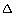ABC,

(ii) The length of perpendicular from A to BC.Solution 3

(i)

Area of the triangle is given by

(ii)

Again area of the triangle

Question 4

The area of an equilateral triangle is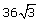sq. cm. Find its perimeter.Solution 4

Question 5

Find the area of an isosceles triangle with perimeter is 36 cm and base is 16 cm.Solution 5

Since the perimeter of the isosceles triangle is 36cm and base is 16cm. hence the length of each of equal sides areHere

It is given that

Let 'h' be the altitude of the isosceles triangle.

Since the altitude from the vertex bisects the base perpendicularly, we can apply Pythagoras Theorem.

Thus we have,

Question 6

The base of an isosceles triangle is 24 cm and its area is 192 sq. cm. Find its perimeter.Solution 6

It is given that

Let 'a' be the length of an equal side.

Hence perimeter=Question 7

The given figure shows a right-angled triangle ABC and an equilateral triangle BCD. Find the area of the shaded portion.

Solution 7

From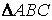,

Area ofArea ofNow

Question 8

Find the area and the perimeter of quadrilateral ABCD, given below; if AB = 8 cm, AD = 10 cm, BD = 12 cm, DC = 13 cm andDBC = 90o

Solution 8

Given , AB = 8 cm, AD = 10 cm, BD = 12 cm, DC = 13 cm andDBC = 90o

Hence perimeter=8+10+13+5=36cm

Area ofArea ofNow

Question 9

The base of a triangular field is three times its height. If the cost of cultivating the field at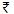36.72 per 100 m2 is49,572; find its base and height.Solution 9

Question 10

The sides of a triangular field are in the ratio 5 : 3 : 4 and its perimeter is 180 m. Find:

(i) its area.

(ii) altitude of the triangle corresponding to its largest side.

(iii) the cost of leveling the field at the rate of Rs. 10 per square metre.Solution 10

(i)

(ii)

Consider the following figure.

(iii)

Question 11

Each of equal sides of an isosceles triangle is 4 cm greater than its height. IF the base of the triangle is 24 cm; calculate the perimeter and the area of the triangle.Solution 11

Let the height of the triangle be x cm.

Equal sides are (x+4) cm.

According to Pythagoras theorem,

Hence perimeter=Area of the isosceles triangle is given by

Here a=20cm

b=24cm

hence

Question 12

Calculate the area and the height of an equilateral triangle whose perimeter is 60 cm.Solution 12

Each side of the triangle isHence the area of the equilateral triangle is given by

The height h of the triangle is given by

Question 13

In triangle ABC; angle A = 90o, side AB = x cm, AC = (x + 5) cm and area = 150 cm2. Find the sides of the triangle.Solution 13

The area of the triangle is given as 150sq.cm

Hence AB=15cm,AC=20cm and

Question 14

If the difference between the sides of a right angled triangle is 3 cm and its area is 54 cm2; find its perimeter.Solution 14

Let the two sides be x cm and (x-3) cm.

Now

Hence the sides are 12cm, 9cm and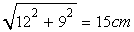The required perimeter is 12+9+15=36cm.Question 15

AD is altitude of an isosceles triangle ABC in which AB = AC = 30 cm and BC = 36 cm. A point O is marked on AD in such a way thatBOC = 90o. Find the area of quadrilateral ABOC.Solution 15

Since AB=AC and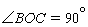Now

## Chapter 20 - Area and Perimeter of Plane Figures Exercise Ex. 20(B)

Question 1

Find the area of a quadrilateral one of whose diagonals is 30 cm long and the perpendiculars from the other two vertices are 19 cm and 11 cm respectively.Solution 1

Question 2

The diagonals of a quadrilateral are 16 cm and 13 cm. If they intersect each other at right angles; find the area of the quadrilateral.Solution 2

Question 3

Calculate the area of quadrilateral ABCD, in which ABD = 90o, triangle BCD is an equilateral triangle of side 24 cm and AD = 26 cm.Solution 3

Consider the figure:

From the right triangle ABD we have

The area of right triangle ABD will be:

Again from the equilateral triangle BCD we have

Therefore the area of the triangle BCD will be:

Hence the area of the quadrilateral will be:

Question 4

Calculate the area of quadrilateral ABCD in which AB = 32 cm, AD = 24 cm A = 90o and BC = CD = 52 cm.Solution 4

The figure can be drawn as follows:

Here ABD is a right triangle. So the area will be:

Again

Now BCD is an isosceles triangle and BP is perpendicular to BD, therefore

From the right triangle DPC we have

So

Hence the area of the quadrilateral will be:

Question 5

The perimeter of a rectangular field is km. If the length of the field is twice its width; find the area of the rectangle in sq. metres.Solution 5

Let the width be x and length 2x km.

Hence

Hence the width is 100m and length is 200m

The required area is given by

Question 6

A rectangular plot 85 m long and 60 m broad is to be covered with grass leaving 5 m all around. Find the area to be laid with grass.Solution 6

Length of the laid with grass=85-5-5=75m

Width of the laid with grass=60-5-5=50m

Hence area of the laid with grass is given by

Question 7

The length and the breadth of a rectangle are 6 cm and 4 cm respectively. Find the height of a triangle whose base is 6 cm and area is 3 times that of the rectangle.Solution 7

Area of the rectangle is given by

Let h be the height of the triangle ,then

Question 8

How many tiles, each of area 400 cm2, will be needed to pave a footpath which is 2 m wide and surrounds a grass plot 25 m long and 13 m wide?Solution 8

Consider the following figure.

Thus the required area = area shaded in blue + area shaded in red

= Area ABPQ +  Area TUDC +  Area A'PUD' +  Area QB'C'T

= 2Area ABPQ + 2Area QB'C'T

=2(Area ABPQ +Area QB'C'T)

Question 9

The cost of enclosing a rectangular garden with a fence all round, at the rate of 75 paise per metre, is Rs. 300. If the length of the garden is 120 metres, find the area of the field in square metres.Solution 9

Perimeter of the garden

Again, length of the garden is given to be 120 m. hence breadth of the garden b is given by

Hence area of the field

Question 10

The width of a rectangular room is of its length, x, and its perimeter is y. Write an equation connecting x and y. Find the length of the room when the perimeter is 4400 cm.Solution 10

Length of the rectangle=x

Width of the rectangle=

Hence its perimeter is given by

Again it is given that the perimeter is 4400cm.

Hence

Length of the rectangle=1400 cm = 14 mQuestion 11

The length of a rectangular verandah is 3 m more than its breadth. The numerical value of its area is equal to the numerical value of its perimeter.

(i) Taking x as the breadth of the verandah, write an equation in x that represents the above statement.

(ii) Solve the equation obtained in (i) above and hence find the dimensions of the verandah.Solution 11

(i)

Length of the verandah=x+3

According to the question

(ii)

From the above equation

Length =3+3=6mQuestion 12

The diagram, given below, shows two paths drawn inside a rectangular field 80 m long and 45 m wide. The widths of the two paths are 8 m and 15 m as shown. Find the area of the shaded portion.

Solution 12

Consider the following figure.

Thus, the area of the shaded portion

=Area(ABCD) + Area(EFGH) - Area(IJKL)…(1)

Question 13

The rate for a 1.20 m wide carpet is Rs. 40 per metre; find the cost of covering a hall 45 m long and 32 m wide with this carpet. Also, find the cost of carpeting the same hall if the carpet, 80 wide, is at Rs. 25. Per metre.Solution 13

First we have to calculate the area of the hall.

We need to find the cost of carpeting of 80 cm = 0.8 m wide carpet, if the rate of carpeting is Rs. 25. Per metre.

Then

Question 14

Find the area and perimeter of a square plot of land, the length of whose diagonal is 15 metres. Given your answer correct to 2 places of decimals.Solution 14

Let a be the length of each side of the square.

Hence

Hence

And

Question 15

The shaded region of the given diagram represents the lawn in the form of a house. On the three sides of the lawn there are flowerbeds having a uniform width of 2 m.

(i) Find the length and the breadth of the lawn.

(ii) Hence, or otherwise, find the area of the flower-beds.Solution 15

Consider the following figure.

(i)

The length of the lawn = 30 - 2 - 2 = 26 m

The breadth of the lawn = 12 - 2 = 10 m

(ii)

The orange shaded area in the figure is the required area.

Area of the flower bed is calculated as follows:

Question 16

A floor which measures 15 m 8 m is to be laid with tiles measuring 50 m 25 cm. Find the number of tiles required.

Further, if a carpet is laid on the floor so that a space of 1 m exists between its edges and the edges of the floor, what fraction of the floor is uncovered?Solution 16

Number of tiles required

Fraction of floor uncovered=Question 17

Two adjacent sides of parallelogram are 24 cm and 18 cm. If the distance between the longer sides is 12 cm; find the distance between the shorter sides.Solution 17

Since

Hence the distance between the shorter sides is 16m.Question 18

Two adjacent sides of parallelogram are 28 cm and 26 cm. If one diagonal of it is 30 cm long; find the area of the parallelogram. Also, find the distance between its shorter sides.Solution 18

At first we have to calculate the area of the triangle having sides, 28cm, 26cm and 30cm.

Let the area be S.

By Heron's Formula,

Area of a Parallelogram = 2 × Area of a triangle

= 2 × 336

= 672 cm2

We know that,

Area of a parallelogram = Height × Base

⇒ 672 = Height × 26

⇒ Height = 25.84 cm

∴ the distance between its shorter sides is 25.84 cm.Question 19

The area of a rhombus is 216 sq. cm. If its one diagonals is 24 cm; find:

(i) Length of its other diagonal,

(ii) Length of its side,

(iii) Perimeter of the rhombus.Solution 19

(i)

We know that

Here A=216sq.cm

AC=24cm

BD=?

Now

(ii)

Let a be the length of each side of the rhombus.

(iii)

Perimeter of the rhombus=4a=60cm.Question 20

The perimeter of a rhombus is 52 cm. If one diagonal is 24 cm; find:

(i) The length of its other diagonal,

(ii) Its area.Solution 20

Let a be the length of each side of the rhombus.

(i)

It is given that,

AC=24cm

We have to find BD.

Now

Hence the other diagonal is 10cm.

(ii)

Question 21

The perimeter of a rhombus is 46 cm. If the height of the rhombus is 8 cm; find its area.Solution 21

Let a be the length of each side of the rhombus.

We know that,

Question 22

The figure given below shows the cross-section of a concrete structure. Calculate the area of cross-section if AB = 1.8 cm, CD = 0.6 m, DE = 0.8 m, EF = 0.3 m and AF = 1.2 m.

Solution 22

The diagram is redrawn as follows:

Here

AF=1.2m,EF=0.3m,DC=0.6m,BK=1.8-0.6-0.3=0.9m

Hence

Question 23

Calculate the area of the figure given below: which is not drawn scale.

Solution 23

Here we found two geometrical figure, one is a triangle and other is the trapezium.

Now

hence area of the whole figure=150+240=630sq.mQuestion 24

The following diagram shows a pentagonal field ABCDE in which the lengths of AF, FG, GH and HD are 50 m, 40 m, 15 m and 25 m respectively; and the lengths of perpendiculars BF, CH and EG are 50 m, 25 m ad 60 m respectively. Determine the area of the field.

Solution 24

We can divide the field into three triangles and one trapezium.

Let A,B,C be the three triangular region and D be the trapezoidal region.

Now

Area of the figure=Area of A+ Area of B+ Area of C+ Area of D

=3900+1250+312.5+2062.5

=7525sq.mQuestion 25

A footpath of uniform width runs all around the outside of a rectangular field 30 m long and 24 m wide. If the path occupies an area of 360 m2, find its width.Solution 25

Let x be the width of the footpath.

Then

Again it is given that area of the footpath is 360sq.m.

Hence

Hence width of the footpath is 3m.Question 26

A wire when bent in the form of a square encloses an area of 484 m2. Find the largest area enclosed by the same wire when bent to from:

(i) An equilateral triangle.

(ii) A rectangle of length 16 m.Solution 26

Area of the square is 484.

Let a be the length of each side of the square.

Now

Hence length of the wire is=4x22=88m.

(i)

Now this 88m wire is bent in the form of an equilateral triangle.

(ii)

Let x be the breadth of the rectangle.

Now

Hence area=16x28=448m2
Question 27

For each trapezium given below; find its area.

(i)

(ii)

(iii)

(iv)

Solution 27

(i)

Again

(ii)

(iii)

For the triangle EBC,

S=19cm

Let h be the height.

(iv)

In the given figure, we can observe that the non-parallel sides are equal and hence it is an isosceles trapezium.

Therefore, let us draw DE and CF perpendiculars to AB.

Thus, the area of the parallelogram is given by

Question 28

The perimeter of a rectangular board is 70 cm. Taking its length as x cm, find its width in terms of x.

If the area of the rectangular board is 300 cm2; find its dimensions.Solution 28

Let b be the breadth of rectangle. then its perimeter

Again

Hence its length is 20cm and width is 15cm.Question 29

The area of a rectangular is 640 m2. Taking its length as x cm; find in terms of x, the width of the rectangle. If the perimeter of the rectangle is 104 m; find its dimensions.Solution 29

Let b be the width of the rectangle.

Again perimeter of the rectangle is 104m.

Hence

Hence

length=32m

width=20m.Question 30

The length of a rectangle is twice the side of a square and its width is 6 cm greater than the side of the square. If area of the rectangle is three times the area of the square; find the dimensions of each.Solution 30

Let a be the length of the sides of the square.

According to the question

Hence sides of the square are 12cm each and

Length of the rectangle =2a=24cm

Width of the rectangle=a+6=18cm.Question 31

ABCD is a square with each side 12 cm. P is a point on BC such that area of ABP: area of trapeziumAPCD = 1: 5. Find the length of CP.Solution 31

Question 32

A rectangular plot of land measures 45 m 30 m. A boundary wall of height 2.4 m is built all around the plot at a distance of 1 m from the plot. Find the area of the inner surface of the boundary wall.Solution 32

Length of the wall=45+2=47m

Breath of the wall=30+2=32m

Hence area of the inner surface of the wall is given by

Question 33

A wire when bent in the form of a square encloses an area = 576 cm2. Find the largest area enclosed by the same wire when bent to form;

(i) an equilateral triangle.

(ii) A rectangle whose adjacent sides differ by 4 cm.Solution 33

Let a be the length of each side.

Hence length of the wire=96cm

(i)

For the equilateral triangle,

(ii)

Let the adjacent side of the rectangle be x and y cm.

Since the perimeter is 96 cm, we have,

Hence

Hence area of the rectangle is = 26 x 22 = 572 sq.cmQuestion 34

The area of a parallelogram is y cm2 and its height is h cm. The base of another parallelogram is x cm more than the base of the first parallelogram and its area is twice the area of the first. Find, in terms of y, h and x, the expression for the height of the second parallelogram.Solution 34

Let 'y' and 'h' be the area and the height of the first parallelogram respectively.

Let 'height' be the height of the second parallelogram

base of the first parallelogram=cm

base of second parallelogram=cm

Question 35

The distance between parallel sides of a trapezium is 15 cm and the length of the line segment joining the mid-points of its non-parallel sides is 26 cm. Find the area of the trapezium.Solution 35

Question 36

The diagonal of a rectangular plot is 34 m and its perimeter is 92 m. Find its area.Solution 36

## Chapter 20 - Area and Perimeter of Plane Figures Exercise Ex. 20(C)

Question 1

The diameter of a circle is 28 cm. Find its:

(i) Circumference

(ii) Area.Solution 1

Let r be the radius of the circle.

(i)

= 88 cm

(ii)

= 616 cm2Question 2

The circumference of a circular field is 308 m. Find is:

(ii) Area.Solution 2

Question 3

The sum of the circumference and diameter of a circle is 116 cm. Find its radius.Solution 3

Let r be the radius of the circle.

Question 4

The radii of two circles are 25 cm and 18 cm. Find the radius of the circle which has circumference equal to the sum of circumferences of these two circles.Solution 4

Circumference of the first circle

Circumference of the second circle

Let r be the radius of the resulting circle.

Question 5

The radii of two circles are 48 cm and 13 cm. Find the area of the circle which has its circumference equal to the difference of the circumferences of the given two circles.Solution 5

Circumference of the first circle

Circumference of the second circle

Let r be the radius of the resulting circle.

Hence area of the circle

Question 6

The diameters of two circles are 32 cm and 24 cm. Find the radius of the circle having its area equal to sum of the areas of the two given circle.Solution 6

Let the area of the resulting circle be r.

Hence the radius of the resulting circle is 20cm.Question 7

The radius of a circle is 5 m. Find the circumference of the circle whose area is 49 times the area of the given circle.Solution 7

Area of the circle having radius 85m is

Let r be the radius of the circle whose area is 49times of the given circle.

Hence circumference of the circle

Question 8

A circle of largest area is cut from a rectangular piece of card-board with dimensions 55 cm and 42 cm. Find the ratio between the area of the circle cut and the area of the remaining card-board.Solution 8

Area of the rectangle is given by

For the largest circle, the radius of the circle will be half of the sorter side of the rectangle.

Hence r=21cm.

Hence

Question 9

The following figure shows a square cardboard ABCD of side 28 cm. Four identical circles of largest possible size are cut from this card as shown below.

Find the area of the remaining card-board.Solution 9

Area of the square is given by

Since there are four identical circles inside the square.

Hence radius of each circle is one fourth of the side of the square.

Area remaining in the cardboard is Question 10

The radii of two circles are in the ratio 3 : 8. If the difference between their areas is 2695 cm2, find the area of the smaller circle.Solution 10

Let the radius of the two circles be 3r and 8r respectively.

According to the question

Hence radius of the smaller circle is

Area of the smaller circle is given by

Question 11

The diameters of three circles are in the ratio 3 : 5 : 6. If the sum of the circumferences of these circles be 308 cm; find the difference between the areas of the largest and the smallest of these circles.Solution 11

Let the diameter of the three circles be 3d, 5d and 6d respectively.

Now

Question 12

Find the area of a ring shaped region enclosed between two concentric circles of radii 20 cm and 15 cm.Solution 12

Question 13

The circumference of a given circular park is 55 m. It is surrounded by a path of uniform width 3.5 m . Find the area of the path.Solution 13

Let r be the radius of the circular park.

Radius of the outer circular region including the path is given by

Area of that circular region is

Hence area of the path is given by

Question 14

There are two circular gardens A and B. The circumference of garden A is 1.760 km and the area of garden B is 25 times the area of garden A. Find the circumference of garden B.Solution 14

Question 15

A wheel has diameter 84 cm. Find how many completer revolutions must it make to cover 3.168 km.Solution 15

Question 16

Each wheel of a car is of diameter 80 cm. How many completer revolutions does each wheel make in 10 minutes when the car is travelling at a speed of 66 km per hour?Solution 16

Question 17

An express train is running between two stations with a uniform speed. If the diameter of each wheel of the train is 42 cm and each wheel makes 1200 revolutions per minute, find the speed of the train.Solution 17

Question 18

The minute hand of a clock is 8 cm long. Find the area swept by the minute hand between 8.30 a.m. and 9.05 a.m.Solution 18

Time interval isArea covered in one 60 minutes=

Hence area swept in 35 minutes is given by

Question 19

The shaded portion of the figure, given alongside, shows two concentric circles.

If the circumference of the two circles be 396 cm and 374 cm, find the area of the shaded portion.

Solution 19

Let R and r be the radius of the big and small circles respectively.

Question 20

In the given figure, the area of the shaded portion is 770 cm2. If the circumference of the outer circle is 132 cm, find the width of the shaded portion.

Solution 20

From the given data, we can calculate the area of the outer circle and then the area of inner circle and hence the width of the shaded portion.

Question 21

The cost of fencing a circular field at the rate of240 per  meter is52,800. The field is to be ploughed at the rate of12.50 per m2. Find the cost of pouching the field.Solution 21

Let the radius of the field is r meter.

Therefore circumference of the field will be: meter.

Now the cost of fencing the circular field is 52,800 at rate 240 per meter.

Therefore

Thus the radius of the field is 35 meter.

Now the area of the field will be:

Thus the cost of ploughing the field will be:

Question 22

Two circles touch each other externally. The sum of their areas is 58 cm2 and the distance between their centers is 10 cm. Find the radii of the two circles.Solution 22

Let r and R be the radius of the two circles.

Putting the value of r in (2)

Hence the radius of the two circles is 3cm and 7cm respectively.Question 23

The given figures shows a rectangle ABCD inscribed in a circle as shown alongside.

If AB = 28 cm and BC = 21 cm, find the area of the shaded portion of the given figure.

Solution 23

Question 24

A square is inscribed in a circle of radius 7 cm. Find the area of the square.Solution 24

Since the diameter of the circle is the diagonal of the square inscribed in the circle.

Let a be the length of the sides of the square.

Hence

Hence the area of the square is 98sq.cm.Question 25

A metal wire, when bent in the form of an equilateral triangle of largest area, encloses an area of 484 cm2. If the same wire is bent into the form of a circle of largest area, find the area of this circle.Solution 25

## Chapter 20 - Area and Perimeter of Plane Figures Exercise Ex. 20(D)

Question 1

The perimeter of a triangle is 450 m and its side are in the ratio 12 : 5 : 13. Find the area of the triangle.Solution 1

Let the sides of the triangle be

a = 12x

b = 5x

c = 13x

Given that the perimeter = 450 cm

⇒ 12x + 5x + 13x = 450

⇒ 30x = 450

⇒ x = 15

Hence, the sides of a triangle are

a = 12x = 12(15) = 180 cm

b = 5x = 5(15) = 75 cm

c = 13x = 13(15) = 195 cm

Now,

Question 2

A triangle and a parallelogram have the same base and the same area. If the side of the triangle are 26 cm, 28 cm and 30 cm and the parallelogram stands on the base 28 cm, find the height of the parallelogram.Solution 2

Let the sides of the triangle be

a = 26 cm, b = 28 cm and c = 30 cm

Now,

Question 3

Using the information in the following figure, find its area.

Solution 3

Construction: Draw CM ⊥ AB

In right-angled triangle CMB,

BM2 = BC2 - CM2 = (15)2 - (9)2 = 225 - 81 = 144

⇒ BM = 12 m

Now, AB = AM + BM = 23 + 12 = 35 m

Question 4

Sum of the areas or two squares is 400 cm2. If the difference of their perimeters is 16 cm, find the sides of the two squares.Solution 4

Let the sides of two squares be a and b respectively.

Then, area of one square, S1 = a2

And, area of second square, S2 = b2

Given, S1 + S2 = 400 cm2

⇒ a2 + b2 = 400 cm2 …..(1)

Also, difference in perimeter = 16 cm

⇒ 4a - 4b = 16 cm

⇒ a - b = 4

⇒ a = (4 + b)

Substituting the value of 'a' in (1), we get

(4 + b)2 + b2 = 400

⇒ 16 + 8b + b2 + b2 = 400

⇒ 2b2 + 8b - 384 = 0

⇒ b2 + 4b - 192 = 0

⇒ b2 + 16b - 12b - 192 = 0

⇒ b(b + 16) - 12(b + 16) = 0

⇒ (b +16)(b - 12) = 0

⇒ b + 16 = 0 or b - 12 = 0

⇒ b = -16 or b = 12

Since, the side of a square cannot be negative, we reject -16.

Thus, b = 12

⇒ a = 4 + b = 4 + 12 = 16

Hence, the sides of a square are 16 cm and 12 cm respectively.Question 5

Find the area and the perimeter of a square with diagonal 24 cm. [Take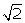=1.41]Solution 5

Diagonal of a square = 24 cm

Question 6

A steel wire, when bent in the form of a square, encloses an area of 121 cm2. The same wire is bent in the form of a circle. Find area the circle.Solution 6

Question 7

The perimeter of a semicircular plate is 108 cm. find its area.Solution 7

Question 8

Two circles touch externally. The sum of their areas is 130π sq. cm and the distance between their centres is 14 cm. Find the radii of the circles.Solution 8

Let the radii of two circles be r1 and r2 respectively.

Sum of the areas of two circles = 130π sq. cm

⇒ πr12 + πr22 = 130π

⇒ r12 + r22 = 130 ….(i)

Also, distance between two radii = 14 cm

⇒ r1 + r2 = 14

⇒ r1 = (14 - r2)

Substituting the value of r1 in (i), we get

(14 - r2)2 + r22 = 130

⇒ 196 - 28r2 + r22 + r22 = 130

⇒ 2r22 - 28r2 + 66 = 0

⇒ r22 - 14r2 + 33 = 0

⇒ r22 - 11r2 - 3r2 + 33 = 0

⇒ r(r2 - 11) - 3 (r2 - 11) = 0

⇒ (r2 - 11) (r2 - 3) = 0

⇒ r2 = 11 or r2 = 3

⇒ r1 = 14 - 11 = 3 or r1 = 14 - 3 = 11

Thus, the radii of two circles are 11 cm and 3 cm respectively.Question 9

Solution 9

Question 10

Two circles touch each other externally. The sum of their areas is 74π cm2 and the distance between their centres is 12 cm. Find the diameters of the circle.Solution 10

Question 11

If a square in inscribed in a circle, find the ratio of the areas of the circle and the square.Solution 11

error: Content is protected !!